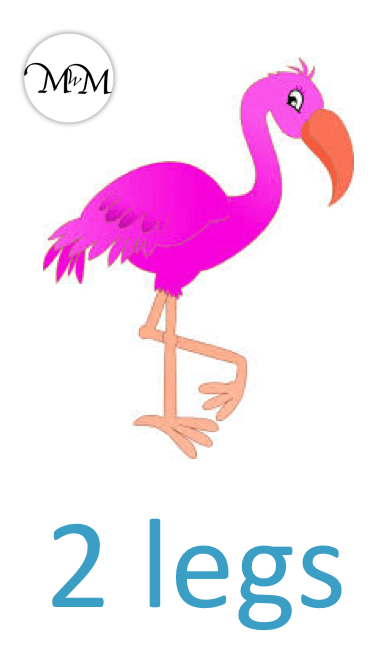• Each flamingo has 2 legs.
• The multiplication sign ‘×’ can mean ‘lots of’
• We can write ‘1 lot of 2’ as ‘1 × 2’.
• We can write 2 + 2 as ‘2 × 2’. This is 2 lots of 2.
• We can write 2 + 2 + 2 as ‘3 × 2’. This is 3 lots of 2.
• We can write 2 + 2 + 2 + 2 as ‘4 × 2’. This is 4 lots of 2.

The multiplication sign ‘×’ can mean ‘lots of’.

The number before the ‘×’ sign tells us how many times we are adding the number after the ‘×’ sign.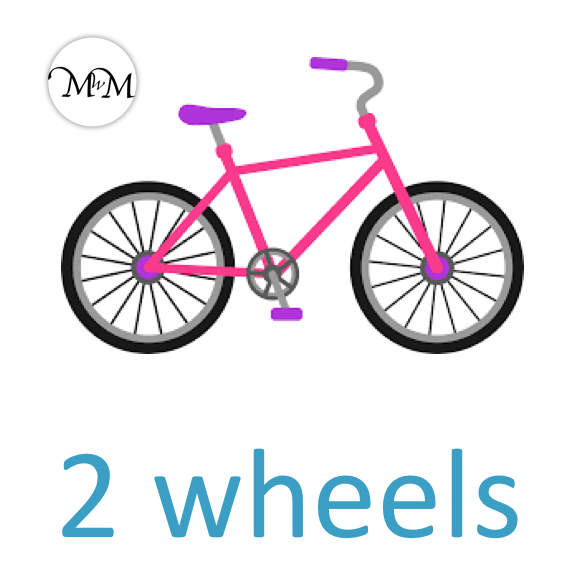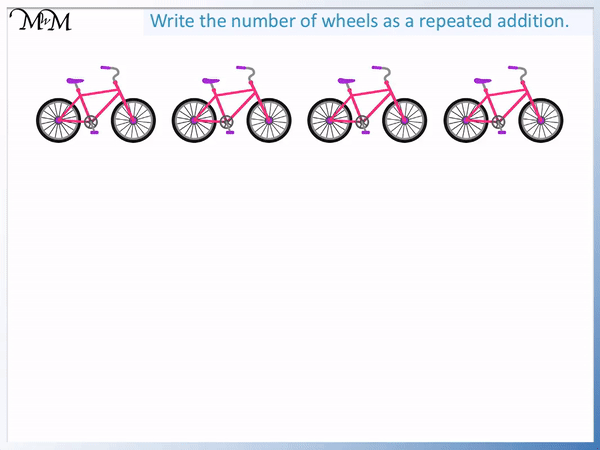• Each bicycle has 2 wheels.
• Here are 4 bicycles.
• To find the total number of wheels, we add them.
• We have 2 + 2 + 2 + 2.
• We have 4 lots of 2.
• The multiplication sign ‘×’, means ‘lots of’.
• 4 ‘lots of’ 2 can be written as 4 × 2.
• We know that 4 × 2 = 8 from our times tables.Supporting Lessons# What is Multiplication as Repeated Addition?

Multiplication is a repeated addition of the same number. The multiplication sign is written as ‘×’.

The number before the multiplication sign tells us how many times we are adding the number that comes after the multiplication sign.

An example of multiplication is 5 × 3. This means 5 lots of 3 or 5 equal groups of 3. It means to add 3 five times.

5 × 3 means 3 + 3 + 3 + 3 + 3. Multiplication is the same as repeated addition.Here are some more examples of multiplication as repeated addition.

Each flamingo has 2 legs.• 1 lot of 2 can be written as 1 × 2.
• 2 lots of 2 can be written as 2 × 2. As repeated addition it means 2 + 2.
• 3 lots of 2 can be written as 3 × 2. As repeated addition it means 2 + 2 + 2.
• 4 lots of 2 can be written as 4 × 2. As repeated addition it means 2 + 2 + 2 + 2.
• We can see that 2 + 2 + 2 + 2 requires us to add 4 lots of 2. This is relatively long to write out and not a quick way to work out the total.

It is quicker to write 4 × 2. Also we know that 4 × 2 = 8 through memorising the times tables.

Multiplication is useful because it is a faster way to do repeated addition and it is easier to write. Learning times tables is important because it allows us to calculate repeated additions immediately and it is necessary for understanding further concepts such as algebra.

Here is an example of writing repeated addition as a multiplication.

Each box of eggs contains 6 eggs.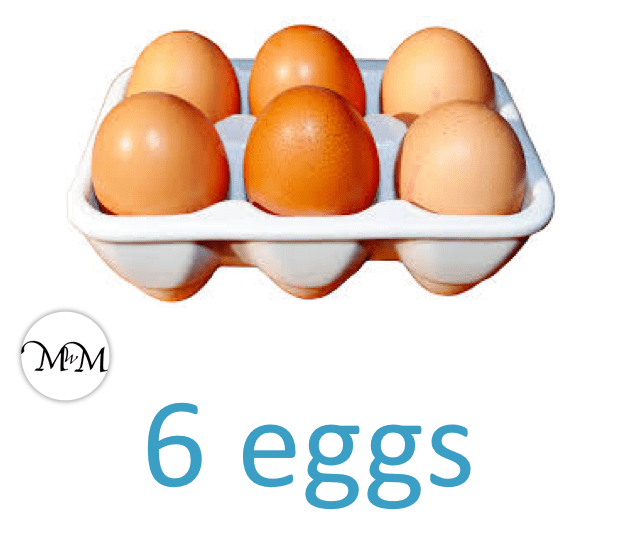Here are 3 boxes of eggs.

To find the total number of eggs in the three boxes, we do an addition.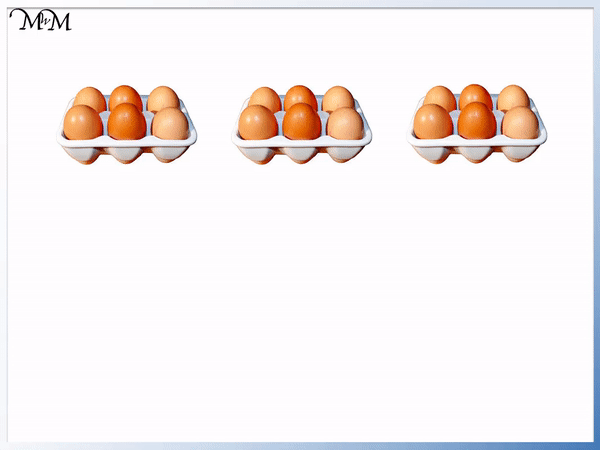We have 6 + 6 + 6 in total. We have 3 sixes added together.

We have 3 lots of 6.

We can write this as 3 × 6.

From our times tables, we should know that 3 × 6 = 18.

There are 18 eggs in total.

Here is another example of multiplication as repeated addition.

Each bicycle has 2 wheels.Here are 4 bicycles.

To find the total number of wheels on 4 bicycles, we will add the number of wheels on each bike to make a total.We have 2 + 2 + 2 + 2.

We have 4 lots of 2.

We can write this repeated addition as 4 × 2. There are 8 wheels in total on 4 bicycles.

Here is another example of multiplication as repeated addition.

Each horse has 4 legs.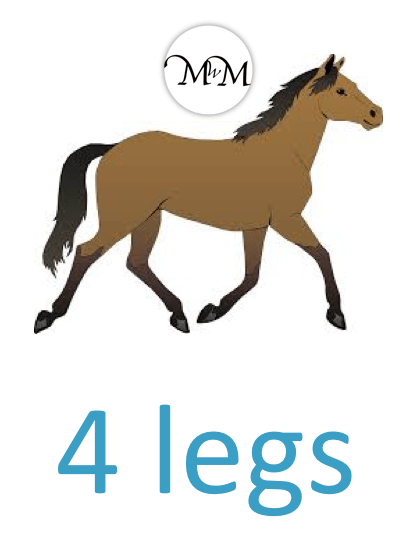We will find the number of legs on 5 horses.We have the repeated addition of 4 + 4 + 4 + 4 + 4.

We have 5 lots of 4.

We ca write this as 5 × 4.

From our times tables, 5 × 4 = 20.

There are 20 legs on 5 horses.

Here is an example where there are 3 cubes in each set.

We have 5 sets of cubes.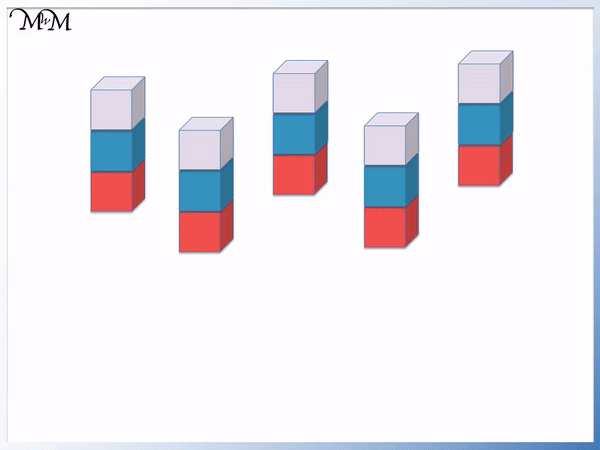In total we have 3 + 3 + 3 + 3 + 3.

We have 5 equal groups of 3.

We can write this as 5 × 3.

From our times tables we know that 5 × 3 = 15.

There are 15 cubes in total.Now try our lesson on Order of Multiplication where we learn rules about the order of multiplication.error: Content is protected !!